# Equations reduced to squares

##### 1. CRIMINAL RATIONAL EQUATIONS WHICH ARE REDUCED TO SQUARE EQUATIONS

An equation in which the variable is contained in the denominator of a fraction is called a fractional rational equation.
When solving fractional rational equations, most often, the procedure is:

– the denominators of multipliers are decomposed;
– the definition area of the equation is determined;
– we get rid of the fractions by multiplying the equation by NHS for the denominators;
– the obtained equation is solved;
– it is determined which of the obtained solutions are solutions of the initial equation.

Example 2. Let's solve the equationThe defining area of the equation is the set

D = R {-2, 2}.

Multiply the equation by (x – 2) (x + 2), so we get the equation:

х (х + 2) – З (х – 2) = 8,

that is, the equation:

х² – х – 2 = 0

whose solutions are х₁ = -1 and х₂ = 2. Since 2 ∉ D, it follows that the equation has only one root x = –1.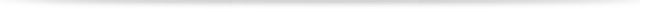##### 2. BINOMINAL EQUATIONS

The equation of type

xⁿ – а = 0

is called the nth degree binomial equation.

With the c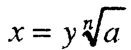hange it comes down to a simpler equation:

уⁿ – 1 = 0

Depending on the coefficient а and the parity of the number n, for the binomial equation х ра – а = 0, the following applies:

1) If a = 0, the equation in the set of real numbers, ie the set of complex numbers has a unique solution x = 0

2) If a ≠ 0, then in the set of real numbers the equation for:

a) n = 2k and a> 0 has two real roots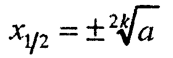b) n = 2k and a <0 has no real roots;

c) n = 2k + 1 and (∀a R) has a single real root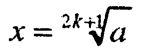3) If a ≠ 0 is an arbitrary real number (or complex) number, then there are exactly n roots in the set of complex numbers.##### 3. BIQUARD EQUATIONS

The equation of type

ах⁴ + bx² + c = 0

where a, b, c are real numbers and a ≠ 0 is called a biquadratic equation.

We solve the biquadratic equation with the help of the change χ² = у which reduces it to the quadratic equation:

ay² + by + c = 0

If the solutions of the equation ay² + by + c = 0 are у₁ and у₂, then based on the change х² = у we get the set of equations:

x² = y₁, x² = y₂

For у₁, у₂ ∈ R, the solutions of the biquadratic equation are:аx⁴ + bx² + c = 0, at c = 0 gets the type:

ax⁴ + bx² = 0 ie x² (ax² + b) = 0

whose solution comes down to solving two incomplete quadratic equations:

x² = 0 and ах² + b = 0.

If b = 0, then the biquadratic equation аx⁴ + bx² + c = 0 is reduced to a fourth degree binomial equation ах⁴ + с = 0.

For this equation, at ace> 0 all four roots are complex, and for ace <0 it has two real and two complex roots.

If b = c = 0, then the biquadratic equation has a quadratic root x = 0.##### 4. TRINOMAL EQUATIONS

The equation of type

ах²ⁿ + bxⁿ + c = 0 where a, b, c ∈ R and а ≠ 0 is called a trinomial equation.

The equation is of the 2nd degree and its solution with the shift

хⁿ = у

comes down to solving the quadratic equation ay² + by + c = 0.

If u and u₂ are the roots of the last equation, then the roots of the trinomial equation are obtained by solving the set of binomial equations:

хⁿ = У₁
хⁿ = У₂##### 5. SQUARE EQUATIONS

These equations usually have the type:

a[ f (x)²] + b [ f (x)]+ c = 0

where a, b, c ∈ R (a ≠ O), and f (x) is an expression that depends on x.
Such equations are called quadratic equations, because the change f (x) = u comes down to a quadratic equation:

ay² + by + c = 0

One root of the third degree symmetric equation is always the number -1, and the other two are obtained by solving the quadratic equation:

ах² + (b – a) x + a = 0

The third degree equation of type

ах³ – bx² – bx – a = 0, (а ≠ 0)

is solved in a similar way as the third degree symmetric equation. The number 1 is always the solution to this equation.###### 6. FOURTH DEGREE SYMMETRIC EQUATION

The equation of type:

ах⁴ + bx³ + сх² + bx + а = о, (а ≠ 0), is called the fourth degree symmetric equation.

By grouping the terms with equal coefficients, we get the equation:

а (х⁴ + 1) + b (x³ + х) + сх² = 0

Divide this equation by x² (x ≠ 0) and get the equation(*)

We introduce a new variable with the shi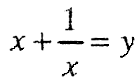ft, from which squaring is obtained: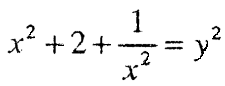that isBy substituting the corresponding values in (*), we get the pawn

а (у² – 2) + by + c = 0

If у₁, у₂ ∈ R are the roots of the last equation, then the values of x are found from the equations:that is, from the set of equations:Hence the four roots of the symmetric equation are obtained, provided that у₁, у₂ ∈ R

The quadratic equation of the type:

ax⁴ + bx³ ± bx – a = 0 (a ≠ 0),

is solved by decomposing its left-hand side into factors, giving:

(х² ± 1) (ах² + bx + a) = 0###### 7. FOURTH DEGREE SYMMETRIC EQUATIONS

The equation of form:

ах⁵ + bx⁴ + сх³ + cx² + bx + а = 0, (a. 0) is a fifth degree symmetric equation.

The solution procedure is as follows:

– the members with the same coefficients are grouped:

a (x³ + 1) + bx (x³ + 1) + cx² (x + 1) = 0

* x³ + 1 = (x + 1) (x² – x + 1) and

* x⁵ + 1 = (x + 1) (x⁴ – x³ + x² – x + 1)

the equation takes the form:

(x + 1)[ ax⁴ + (b-a)x³ + (a – b + c)x² + (b-a) x + a] = 0

One root is x₁ = -1, and the other 4 roots are obtained by solving the fourth degree symmetric equation:

ax⁴ + (b-a) x³ + (a – b + c) x² + (b-a) x + a = 0

The fifth degree equation of the type:

ах⁵ + bx⁴ + сх³ + cx² – bx – а = 0, (a ≠ 0),

is solved in a similar way as the fifth degree symmetric equation. One solution to this equation is x = 1. This equation is called the fifth degree obliquely symmetric equation.

– equations that are squared –

Total Page Visits: 319 - Today Page Visits: 1